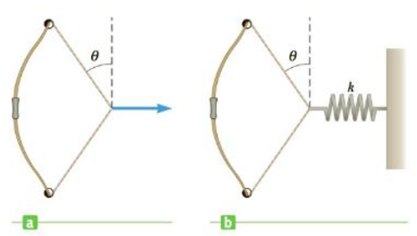Chapter 13, Problem 6P

Chapter
Section
Textbook Problem

An archer must exert a force of 375 N on the bowstring shown in Figure P13.6a such that the siring makes an angle of θ = 35.0° with the vertical. (a) Determine the tension in the bowstring. (b) If the applied force is replaced by a stretched spring as in Figure P13.6b and the spring is stretched 30.0 cm from its unstretched length, what is the spring constant?Figure P13.6

a)

To determine
The tension in the bowstring.

Explanation

Given info: The force applied on the bow string F   is 375 N. The angle made by the string with the vertical is 350 .

Explanation:

The free body diagram is given below.

In the diagram, the vertical components Tcos35° cancel each other. The horizontal components Tsin35° add up to give 2Tcos35° which is balanced by the applied force F

b)

To determine
The spring constant of the stretched spring which replaces the 375 N force applied.

Still sussing out bartleby?

Check out a sample textbook solution.

See a sample solution

The Solution to Your Study Problems

Bartleby provides explanations to thousands of textbook problems written by our experts, many with advanced degrees!

Get Started

Find more solutions based on key concepts